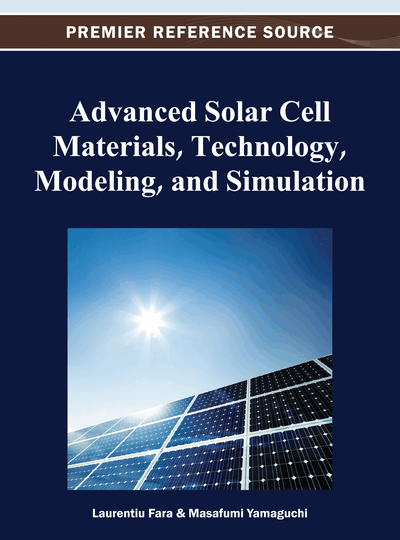# Phononic Engineering for Hot Carrier Solar Cells

Sana Laribi (Institute of Research and Development on Photovoltaic Energy (IRDEP), France), Arthur Le Bris (Institute of Research and Development on Photovoltaic Energy (IRDEP), France), Lun Mei Huang (Institute of Research and Development on Photovoltaic Energy (IRDEP), France), Par Olsson (Institute of Research and Development on Photovoltaic Energy (IRDEP), France) and Jean Francois Guillemoles (Institute of Research and Development on Photovoltaic Energy (IRDEP), France)
DOI: 10.4018/978-1-4666-1927-2.ch012

## Abstract

In this chapter, the authors first analyse the operation of a hot carrier solar cell and lay down the general principles. They then discuss the opportunity of phonon engineering to improve the phonon bottleneck. Finally, they present how these can be modeled in nanostuctures comprising several thousand atoms, where true 3D phonon dispersion relations for Si-Ge nano-structures are obtained using first principles methods. The effects of the nano-structure size and geometry on the phonon dispersion relations are investigated. The possible phonon decay processes in the nano-structures are discussed and compared with the bulk crystal materials. The performance of calculated nano-structures on the hot carrier solar cell is evaluated with the acquired knowledge of phonon modes.
Chapter Preview
Top

## 1. The Hot Carrier Solar Cell Concept

### 1.1. Principles of the Hot Carrier Solar Cell

The concept of hot carrier solar cell was first introduced by Ross and Nozik (1982). In standard devices, the photon energy in excess of the threshold absorption energy is given to the photogenerated carrier population, and then to the lattice as heat, with only the band gap energy being converted as electrical energy. If the carrier thermalization (thermal equilibration of carriers with the lattice) is suppressed, then the conversion of the total available energy into potential energy is possible and leads to higher conversion efficiency.

The energy distribution of the photogenerated carriers is, immediately after absorption, a non-equilibrium distribution that depends on the energy distribution of the incident photons, and on the electron and hole effective masses and density of states. After absorption, the fate of the carrier distribution can be:

• Hot Non-Equilibration: The carriers are not even equilibrated among themselves, the carrier temperature cannot be defined.

• Hot Equilibration: The carriers equilibrate among themselves but not with the lattice, resulting in a hot carrier population at TH > TC, where TC is the temperature of the lattice.

• Full Thermalization: The common situation where carriers are at thermal equilibrium with the environment at ambient temperature.

The comparison between the thermalization rate (due to interactions with phonons, see section 1.3), the carrier-carrier scattering rate, and the carrier extraction rate (the rate at which carriers are removed from the system through contacts or by radiative recombination) will determine which one of the above cases applies.

Typical time constants for these different mechanisms in III-V materials are given in Table 1, from Othonos (1998).

Table 1.
Typical time constants of various carrier interaction mechanisms in semiconductors, from Othonos (1998)
 Type of interaction Characteristic time (s) Carrier-Carrier scattering 10-15 – 10-12 Carrier - Optical phonon interaction ≥ 10-12 Optical phonon - Acoustic phonon interaction ~ 10-11 Auger recombination ~ 10-10 Radiative recombination ≥ 10-9

The carrier-carrier scattering time constant is usually below the picosecond, and decreases with an increasing carrier density, while the time constant for the carrier-phonon interaction is of the order of the picosecond. Considering these time constants and without carrier extraction, the dynamics of the carrier after a pulsed monochromatic excitation is described in Figure 1 from Green (2003). Immediately after absorption at t = 0, carriers are generated in a narrow energy range (2). Carrier-carrier scattering then occurs and carriers are redistributed in a hot thermal distribution (3)-(4), within a picosecond. This distribution is then cooled towards the lattice temperature because of interaction with phonons (5). Finally, carriers recombine with a sub-microsecond time constant (7).

If the excitation rate is higher than the thermalization rate, and lower than the carrier-carrier scattering rate, a steady state hot distribution can be established, and the carrier kinetic energy, which is usually lost as heat, can be used and contributes to the conversion efficiency.

Assuming such steady state hot carrier population is achieved, a specific care is required regarding carrier extraction. If the photogenerated electrons and holes are withdrawn towards metallic electrodes, where interactions with the lattice cannot be avoided before they produce work in the external circuit, thermalization of excited carriers to the lattice temperature will occur, and with it, the carrier kinetic energy will be lost.

A specific cooling of the carriers with minimum heat losses (adiabatic cooling) is necessary to cool down the carriers to the lattice temperature with production of chemical energy that appears as quasi-Fermi level splitting. In other words, the carrier’s kinetic energy has to be converted into potential energy, or voltage. This can be achieved with energy selective contacts (Würfel, 1997) that are described in Section 1.4.

In Figure 2, a schematic of the principle of a hot carrier solar cell is presented. Photons are absorbed in the absorber having a band gap Eg, where carriers are in thermal and chemical disequilibrium with the lattice at temperature TC, characterized by a temperature TH > TC and a quasi Fermi level splitting ΔµH = µe - µh ≠ 0. Carriers are extracted through selective contacts with a transmission energy range δE, electrons at energy Eeext, and holes at energy Ehext, towards electrodes with an applied voltage V = (µn - µp) / q. The extraction energy Eext is defined as the difference EeextEhext. Eext can be higher than the band gap.

## Complete Chapter List

Search this Book:
Reset